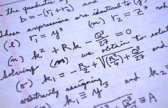# Do the Math

Photomath. 10 Math Apps that Teach Number Concepts. By Katie Chirhart When you type “math” into the Apple App Store, the majority of apps found focus on math facts.These are great for children to do at home or at school for additional practice. However, there are a wide variety of apps that focus on teaching the concepts behind mathematical processes—a critical piece of the learning equation. The beauty of these apps is often overlooked. They allow students to play and experiment with mathematical concepts in ways they cannot do with physical manipulatives. While many of them are not free, if you purchase these math apps for a teacher iPad and use reflecting software, they are worth the expense. 1.

This amazing app starts by focusing on simple one-to-one correspondence and number recognition. It’s an engaging app that’s perfect for kinders and first graders. 2. In my opinion, this app was designed specifically for teachers and includes important letter and phoneme options, as well as mathematical options. 3. 4. 5. 6. 7. 8. 9. 5 Online Calculators to Improve Your Basic Math Skills. The first “compact” calculator was released in 1957, and needed to be built into a desk.Over time the device shrank, to the point where you could easily fit it in your hand. These days most people use software calculators on their phones, instead of a separate device. 10 Free Math Games Your Kids Should Be Playing. The mere mention of the word “mathematics” is enough to strike fear into the hearts of adults around the world.For thousands of people, the thought of doing annual tax returns, applying for a mortgage, or even just helping children with their homework can bring them out in an episode of cold sweats and get them running for the nearest calculator. Luckily their are phone apps to help adults improve, but the long-term solution appears reasonably obvious; children need to be engaged with maths from a young age, making use of tools, games, and apps that make the process of learning arithmetic fun rather than an arduous task.

Here we look at some of the best games to help children learn mathematics in a fun way: Fraction Flags (Ages 7-9) Learn Math. Mathematics Stack Exchange. MacTutor History of Mathematics. Statistics Problems With Solutions. Problem 1 In one state, 52% of the voters are Republicans, and 48% are Democrats.In a second state, 47% of the voters are Republicans, and 53% are Democrats. Suppose a simple random sample of 100 voters are surveyed from each state. What is the probability that the survey will show a greater percentage of Republican voters in the second state than in the first state? Solution The correct answer is C. The solution involves four steps. Make sure the sample size is big enough to model differences with a normal population. Therefore, the probability that the survey will show a greater percentage of Republican voters in the second state than in the first state is 0.24. Statistics 101. AP Calculus AB-BC - Functions, Graphs, & Limits - Limits of Functions to a Constant - (2x^2-4x-2)/(x^2-4) 17Calculus - You CAN Ace Calculus. Calculus Help. Practice Trigonometry. Free Trigonometry Practice Tests. Our completely free Trigonometry practice tests are the perfect way to brush up your skills.Take one of our many Trigonometry practice tests for a run-through of commonly asked questions. You will receive incredibly detailed scoring results at the end of your Trigonometry practice test to help you identify your strengths and weaknesses. Pick one of our Trigonometry practice tests now and begin! High school Trigonometry classes introduce students to various trigonometric identities, properties, and functions in detail. Students typically take Trigonometry after completing previous coursework in Algebra and Geometry, but before taking Pre-Calculus and Calculus.Trigonometry for you Your background How to learn trigonometry Applications of trigonometry Astronomy and geography Engineering and physics Mathematics and its applications What is trigonometry? Trigonometry as computational geometry Angle measurement and tables Background on geometry The Pythagorean theorem An explanation of the Pythagorean theorem Similar triangles Angle measurement The concept of angle Radians and arc length Exercises, hints, and answers About digits of accuracy Chords What is a chord? Welcome to Math Test Practice. Geometry of Circles, Triangles, Quadrilaterals, Trapezoids, Proofs and more... Online maths practice and lessons. Algebra 1. Free Online Math Practice Tests!

Prealgebra at Cool math .com: Free Pre-Algebra Lessons and Practice Problems. Pre-Algebra. Arithmetic Game – Online Speed Drill. Arithmetic Practice. MathABC: Online math practice. MATH ADDITION GAME FOR KIDS. Gapminder: Unveiling the beauty of statistics for a fact based world view. Math Problem Solver. Cymath - Math Solver with Steps. Graphing Stories - 15 seconds at a time.

Beautiful, Free Math. GeoGebra. Numberphile. Mathematics Illuminated. Street-Fighting Math. *Note - This is an Archived course* This is a past/archived course.At this time, you can only explore this course in a self-paced fashion. Certain features of this course may not be active, but many people enjoy watching the videos and working with the materials. Make sure to check for reruns of this course. Too much mathematical rigor teaches rigor mortis: the fear of making an unjustified leap even when it lands on a correct result. You will learn this skill by mastering six reasoning tools---dimensional analysis, easy cases, lumping, pictorial reasoning, taking out the big part, and analogy. Your learning will be supported by regular readings that you discuss with other students, by short tablet videos, by quick problems to help you check your understanding, by weekly homework problems, review and and a final exam.

All required readings are available within the courseware, courtesy of The MIT Press. Natural Math. Get The Math. Home - Maths Careers.